Tree is a hierarchical data structure which stores the information naturally in the form of hierarchy style.

Each node is connected to a number of nodes with the help of pointers (edges).

It is a non-linear data structure compared to arrays, linked lists, stack and queue.

Root:Root is a special node in a tree. The entire tree is referenced through it. It does not have a parent.

Parent Node:Parent node is an immediate predecessor of a node.

Child Node:All immediate successors of a node are its children.

Siblings:Nodes with the same parent are called Siblings.

Path:Path is a number of successive edges from source node to destination node.

Height of Node:Height of a node represents the number of edges on the longest path between that node and a leaf.

Height of Tree: Height of tree represents the height of its root node.

Depth of Node:Depth of a node represents the number of edges from the tree's root node to the node.

Degree of Node:Degree of a node represents a number of children of a node.

Edge:Edge is a connection between one node to another. It is a line between two nodes or a node and a leaf.

### Binary Tree

A Tree is said to be a Binary Tree if all of its nodes have atmost 2 children. That is, all of its node can have either no child, 1 child or 2 child nodes.### Properties of a Binary Tree:

1)The maximum number of nodes at level 'l' of a binary tree is (2l - 1). Level of root is 1.
This can be proved by induction.
For root, l = 1, number of nodes = 21-1 = 1
Assume that the maximum number of nodes on level l is 2l-1.
Since in Binary tree every node has at most 2 children, next level would have twice nodes, i.e. 2 * 2l-1.

2)Maximum number of nodes in a binary tree of height 'h' is (2h – 1).
Here height of a tree is the maximum number of nodes on the root to leaf path. The height of a tree with a single node is considered as 1.
This result can be derived from point 2 above. A tree has maximum nodes if all levels have maximum nodes. So maximum number of nodes in a binary tree of height h is 1 + 2 + 4 + .. + 2h-1. This is a simple geometric series with h terms and sum of this series is 2h – 1.
In some books, the height of the root is considered as 0. In this convention, the above formula becomes 2h+1 – 1.

3)In a Binary Tree with N nodes, the minimum possible height or the minimum number of levels is Log2(N+1). This can be directly derived from point 2 above. If we consider the convention where height of a leaf node is considered as 0, then above formula for minimum possible height becomes Log2(N+1) – 1.

4)A Binary Tree with L leaves has at least (Log2L + 1) levels. A Binary tree has maximum number of leaves (and minimum number of levels) when all levels are fully filled. Let all leaves be at level l, then below is true for number of leaves L.
L   <=  2l-1  [From Point 1]
l =  Log2L + 1
where l is the minimum number of levels.

5)In a Binary tree where every node has 0 or 2 children, number of leaf nodes is always one more than nodes with two children.
L = T + 1
Where L = Number of leaf nodes
T = Number of internal nodes with two children

### Types of Binary Trees:

Full Binary Tree: A Binary Tree is full if every node has either 0 or 2 children. The following are examples of a full binary tree. We can also say a full binary tree is a binary tree in which all nodes except leaves have two children.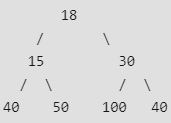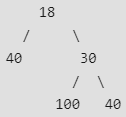In a Full Binary, number of leaf nodes is number of internal nodes plus 1.

Complete Binary Tree: A Binary Tree is complete Binary Tree if all levels are completely filled except possibly the last level and the last level has all keys as left as possible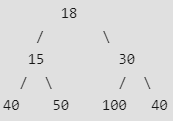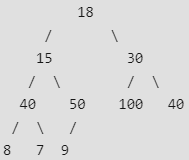Perfect Binary Tree: A Binary tree is Perfect Binary Tree in which all internal nodes have two children and all leaves are at the same level.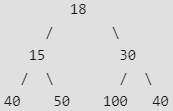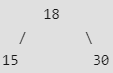A Perfect Binary Tree of height h (where height is the number of nodes on the path from the root to leaf) has 2h – 1 node.

darkmode